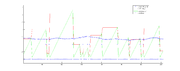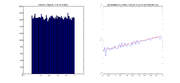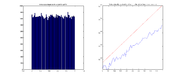# Spike generation using a Poisson process

Retinal ganglion cell (RGC) spike generation in vismodel is implemented in rgcCalculateSpikesByLayer function, called by rgcComputeSpikes. The spike generation uses a Poisson process with time-dependent intensity. We describe the calculations and logic here.

#  What is a Poisson process?

A stochastic process is a finite or infinite sequence of random variables, that represent a probabilistic experiment. A Poisson process is a specific case of this.

In our case, the experiment is sampling spike times randomly. The random variables are the successive spike times, $u_k$, that form a sequence over time.

The Poisson process is a simple process to study and generate because the events occur independently of one another. (A very common example of a Poisson process is the arrival times of customers in the line at the supermarket : they arrive independently of each other. The fact that a customer has just arrived gives no information on when the next one will arrive.) We are making the assumption that spikes arrive independently of one another.

The rate at which arrivals occur in the process is called the intensity or arrival rate (or spike rate in our case), usually called $\lambda$. This rate can be understood like the average number of spike arrivals per unit of time. The bigger $\lambda$, the more often spikes will arrive, so the shorter the time between two spikes becomes.

In more mathematical terms, there are two interesting random variables that can be studied in such a process (apart from the arrival times $u_k$).

• The first is the number of arrivals in a given time interval $[t_1,t_2]$, called $N_{t_1,t_2}$. This follows a Poisson distribution of parameter $\lambda (t_2-t_1)$ : $P(N_{t_1,t_2} = n) = e^{- \lambda (t_2 - t_1)} \frac{(\lambda (t_2 - t_1))^n}{n!}$.
• The second is the time of first arrival, $\tau_1$. It follows an exponential distribution of parameter $\lambda$, that is : $P(\tau_1 \leq t) = 1- e^{- \lambda t} = \int_0^t{\lambda e^{- \lambda t}dt}$. Because the arrivals are independent, this is also the time before the next arrival, whatever the number of arrivals that happened before : $P(\tau_k \leq t | u_{k-1} = t_{k-1}) = P(\tau_1 \leq (t - t_{k-1}))$.

If $\lambda$ is a constant, the process is called homogeneous. The process we will deal with is non-homogeneous : the intensity is a function of time, $\lambda (t)$. It varies with the inputs to the RGC, caused by the visual stimulus and the subsequent activity in the neuron network.

#  Code explanation

##  Membrane Potential

The membrane potential grows over time, due to the inputs coming from (1) the absorptions of the cones, (2) the feedback current that neurons induce upon themselves after a spike, and (3) the coupling current that neighboring spiking neurons induce in a neuron.

(1) The RGCs have a response to the cone absorption stimulus that depends both of space and time. We consider that the reponse is a convolution of the absorptions with a space-time linear filter. First we compute the convolution of absorptions with the spatial filter or Receptive Field of the RGCs in rgcCalculateConvByLayer. Then we compute a time convolution with the time response of each neuron in rgcCalculateTSByLayer (taking eye movements into account). The output of this is the stimulus-induced input to the RGC, called linear time series (linTS in the code) for each neuron.

(2) When a neuron spikes, it starts a feedback time response electrical current, called fbTR in the code, of a given duration in time. This feedback starts being added to the RGCs input directly after a spike, and until the end of the fbTR function. Several feedbacks can be taking place at the same time for a given RGC, if the spikes were frequent enough.

(3) When a neuron spikes, it starts a coupling time response current in its neighbors' input, called cpTR in the code. This works just like the feedback except it is added to neighboring neurons' input instead of the spiking neuron's. The number of neighbors and the strength of the connexion with them is defined by the connexion matrix.

The stimulus-induced input, linTS, is precomputed before spiking. The spike-induced currents (feedback and coupling) are added as the spikes are generated, and added in the time series variable spkTS.

We have a membrane potential $rgcV = linTS + spkTS + randV$, with randV a gaussian voltage of mean rgcP.meanV and standard deviation rgcP.stdV.

• TODO
• Why do we have these values for rgcP.meanV and rgcP.stdV, where do they come from? Is randV a voltage, a current, what does is correspond to in real neurons? Why add a random component here when the spiking is already a random process?
• Does rgcV really correspond to a membrane potential? Does it have units? Are the inputs from linTS and spkTS currents or voltages? If they are currents, how do we convert them to voltages to add to the membrane potential?

##  Spike rate

Pillow has shown that a good model for the spike rate $\lambda (t)$ is an exponential non-linear function of the inputs to the RGC :

$\lambda (t) = e^{linTS(t) + spkTS(t)}$.

• Note : In the old code, we used $\lambda (t) = e^{rgcV}$, with $rgcV = \sum_t{linTS(t)+spkTS(t)}$. We used an "integrated" value of the inputs to the cell.

Experimentally, if we use this formula we get a spike rate of 1 or 2 spikes per second, so most neurons won't spike in a simulation of 200ms. We must add a gain to the spike rate: $\lambda = K e^{linTS + spkTS}$. Pillow doesn't use this probably because he doesn't have any particular units for things.

• Note : This equivalent to having : $\lambda = e^{ln(K)} e^{linTS + spkTS} = e^{ln(K) + linTS + spkTS}$. Therefore, with no stimulus input and no spiking, we should get $\lambda = e^{ln(K)} = K$, the spontaneous spike rate. This could be a way of tuning K : ln(K) is the 'spontaneous input'.
• TODO : our scaling is very arbitrary. we should also add gains in front of linTS and spkTS, and use $\lambda = K e^{K_1*linTS + K_2*spkTS}$. Equivalently, we could add gains to the filter functions (RFs and inTR for producing linTS, fbTR and cpTR for producing spkTS). So far, the filter functions are arbitrarily scaled. In real neurons, linTS and spkTS represent real currents, so we should check (1)what their intensity is, and (2)what their relative intensity is: is the spiking driven more by the stimulus or by the feedback and coupling? If we can't get measures of this, then looking at the relative size of Pillow's filter functions could be a good start for (2), using $\lambda = K e^{K_1(linTS + K_2spkTS)}$ or $\lambda = K e^{K_1(K_2linTS + spkTS)}$, or even just $\lambda = K e^{K_2linTS + spkTS}$. For now, there is a big gain on absorptions : gain = adaptGain*1e6*300/mLum. We should get rid of it before we start tuning the filter functions. We should also tune the relative weight of feedback and coupling (that make up spkTS), or at least imitate Pillow's relative weights.

Values : in the steady state, linTS oscillates on [0.1;0.6] and spkTS in [-0.03;0.07], so the spike rate is largely driven by the stimulus input (in absolute value, linTS is over 85% of the input.). There is a script called testing_rgcPlots for visualizing this and other things.

##  Spike generation

We generate a Poisson process with time-varying rate parameter $\lambda (t)$.

For this Pillow uses the time-rescaling theorem. This states that if the spike arrival times $u_k$ follow a Poisson process of instantaneous rate $\lambda (t)$, then the time-scaled random variables $\Lambda_k = \int_0^{u_k} \lambda (u) du$ form a homogeneous Poisson process of intensity 1. That is, if we consider the $\Lambda_k$ as a new sequence of arrival times, then these arrivals occur independently, and with an average rate of one per time unit.

Therefore, Pillow generates the inter-arrival times $\tau_k$ of the time-scaled process (this is easy : they are independent exponential variables of parameter 1, see #What is a Poisson process?), and from them he deduces the spike arrival times for the original process, $u_k$, using the realtion : $\tau_k = \Lambda_k - \Lambda_{k-1} = \int_{u_{k-1}}^{u_k} \lambda (u) du$.

• Note : It does not make sense to tune the mean of the exponential variables, they should be exactly 1.

The algorithm is as follows, to generate spikes in a total time interval of $[0,T_{end}]$ :

1 . Set $u_0=0$ and k=0.
2 . While $u_k < T_{end}$ :
(i) k = k+1
(ii) Draw $\tau_k$ from an exponential distribution with parameter 1.
(iii) Find the corresponding $u_k$ such that $\tau_k = \int_{u_{k-1}}^{u_k}{\lambda (u) du}$.


Step 2.(iii) is done by incrementing gradually $\int_{u_{k-1}}^{t}{\lambda (u) du}$ over time, until it reaches a value of $\tau_k$, at which point we know we have reached $t=u_k$, the $k^{th}$ spike time.

Since the simulation has discrete time steps, the algorithm becomes :

1 .(i) Set $u_0=0$ and k=0.
(ii) Divide the time into small intervals of length $\delta t$.
2 . While $u_k < T_{end}$ :
(i) k = k+1
(ii) Draw $\tau_k$ from an exponential distribution with parameter 1.
(iii) integral = 0
(iv) for t from $u_k$ to $T_{end}$, in increments of $\delta t$:
integral = integral + $e^{linTS(t) + spkTS(t)} \delta t$
if integral > $\tau_k$ : this is the $k^{th}$ spike time
$u_k$ = t
Break the for loop.


In this way, the integral $\int_{u_{k-1}}^{t}{\lambda (u) du}$ is approached by $\sum_{u = u_{k-1}}^{t}{\lambda (u) \delta t}$, with $\lambda (u) = e^{linTS(u) + spkTS(u)}$.

• Note : this is what the old code did :

1 - We sum up the inputs to the RGC into a rgcV variable : $rgcV(t) = \sum_{u=u_{k-1}}^{t}{linTS(u) + spkTS(u)}$. This is not an integration because we don't multiply by $\delta t$.

2 - We compare the exponential of rgcV, multiplied by $\delta t$ (and divided by 1000 to convert $\delta t$ to seconds), to the exponential variable :

$e^{rgcV(t)} \delta t > \tau_k$

$e^{\sum_{u=u_{k-1}}^{t}{linTS(u) + spkTS(u)}} \delta t > \tau_k$

$\left( \prod_{u=u_{k-1}}^{t}{e^{linTS(u) + spkTS(u)}} \right) \delta t > \tau_k$ ---- instead of ----- $\left( \sum_{u=u_{k-1}}^{t}{e^{linTS(u) + spkTS(u)}} \right) \delta t > \tau_k$ in Pillow's code.

3 - Our $\tau_k$ is sampled from an exponential distribution of parameter $\lambda = mExp = 0.04$ and not 1. The value of mExp was tuned experimentally to give acceptable spikes.

##  Spike-induced signals

When we reach $t=u_k$, the neuron spikes. We start a new feedback signal and add it to the spkTS of the neuron, and start a new coupling signal and add it to the spkTS of neighboring neurons, with a gain defined in the connexion matrix. The further away a neighbor is, the smaller the gain. This part of the code takes the longest in running time (because it's not matrixified, to avoid using too much memory space...), so a given neuron has neighbours no farther than 3 gridspaces away so that it has few neighbors.

Our coupling and feedback functions don't have the same shape as Pillows, because Pillow uses cosine bumps with many parameters, which is too hard to tune (see the script testing_cosineBase.m for visualizing this, it's not that complicated.) Ours have simpler equations.

• Their duration is rgcP.trDur, in ms (default = 100ms). The sampling is done every dT.
• The coupling is A*1/t, with A=linF/tShift, two parameters in rgcP.
• The feedback is a sum of a dip and 2 gamma functions.
• The dip is -A*1/t (opposite of the coupling).
• The gamma functions are $\gamma_i = \frac{1}{\gamma(g_i) b_i}(\frac{t}{b_i})^5 e^{-\frac{t}{b_i}}$, where $b_i$ determines the peak position. We have

$fbTR = dip + f_1 {\gamma}_1 - f_2 {\gamma}_2$ (default : f1=1, f2=0.5).

It is then normalized so that its norm is rgcP.normF.

• TODO : investigate the shape of the response functions. The coupling function cpTR is simple, but the feedback fbTR is NOT.
• why is A = linF/tShift? why use two variables? (tShift is not a time shift in practice, is there a mistake there?)
• The gamma functions in fbTR are used only in $\gamma(g_i)$, in one single point. The shape of the bumps does NOT come from the gamma functions, but from $t^5 e^{-t}$. The gamma functions are uselessly complicated gain values, and they are lost in the renormalization!!
• We could add a refractory period : a flat negative zone in the first time steps of the feedback function. (is this really needed?)

#  Refresh rate

Pillow uses a weird factor called RefreshRate, whose value is fixed at 100. He uses it to divide the integral, which gives :

integral = integral + $e^{linTS(t) + spkTS(t)} \delta t / RefreshRate$

So in the end he is actually comparing : $\frac{1}{RefreshRate} \sum_{u = u_{k-1}}^{t}{\lambda (u) \delta t} > \tau_k$

The RefreshRate is used all over his code, but there is no definition of it (I grepped but didn't find any). It might be that in Pillow's code, RefreshRate is actually 1/K.

#  Results and Discussion

When I add a gain spikeRateGain=K=100 to the spike rate, for 500 time frames, I get this : (see testing_rgcPlots.m for plotting these figures)

We see more spikes in the lighter colors.

 File:ResultsGain100 inputHistogram.png Histogram of rgcV values (inputs to the cell : linTS+spkTS)

The second plot is the spiking probability as a function of the inputs to the cell, rgcV. The experimental spiking probability (in blue) is computed by counting the spikes occurring in each bin of the rgcV input. The red line is the theoretical spiking probability of the Poisson process : $proba(dT) = \lambda(t)*dT + o(dT)$, and the o(dT) is negligible if dT is small enough. Since $proba(t) = \lambda(t)*dT = K*e^{rgcV}*dT = e^{ln(K*dT)+rgcV}$, we should get a straight line on a log plot.Behavior of one neuron over time (the spike rate is normalized by K to fit in the figure)

##  Influence of sampling time

For the Poisson process, the sampling time should be small enough so that the approximations used in our discrete algorithm are admissible.

When plotting the spiking probability as a function of rgcV, we see that taking a bigger dT makes the experimental curve decrease compared to the theoretical one :Spiking probability, with 100,000 uniform samples in [0,1] as input, with dT = 1 msSpiking probability, with 100,000 uniform samples in [0,1] as input, with dT = 6 ms

Possible explanations :

• We have in theory : $proba(dT) = \lambda(t)*dT + o(dT)$, so if dT is too big, the o(dT) is not negligible. Maybe this is happening here.
• At every dT, we check if the integral has overrun the random spike time (see the behavior plots : the integral in green overruns the spike time in red). If dT is big, we will detect the overrun with a delay. So there will be a delay before spiking, and then the process will be reinitialized (new random spike time, integral to zero). Because of this delay, there will be less spikes overall. So the spiking probability is lower.
• Sometimes the time between two spikes is very short. If dT is too big, that spike would be "skipped" altogether.

Is this a problem? How does it impact the information contained in the spike output? Does it impact the classification performance?

• TODO : Maybe dT<<lambda. Try to measure if this is true. (should not be too hard with plots from testing_rgcPlots.m)
• Note : for the contrast and frequency sensitivity experiments, we need to use bigger dT (dT = 4ms works well). So we will have to face this problem.